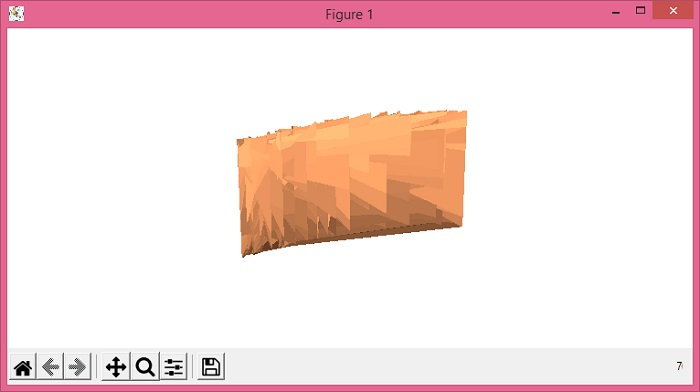# How to surface plot/3D plot from a dataframe (Matplotlib)?

To surface plot/3d, we would require 2D data points, not 1D dataframe.

## Steps

• Set the figure size and adjust the padding between and around the subplots.

• Create a new figure or activate an existing figure using figure() method.

• Add an '~.axes.Axes' to the figure as part of a subplot arrangement using add_subplot() method.

• Initialize a variable 'n' for the number of samples.

• Create x, y and z data points using numpy.

• Use plot_surface() method to make surface 3d.

• To display the figure, use show() method.

## Example

import numpy as np
from matplotlib import pyplot as plt

plt.rcParams["figure.figsize"] = [7.50, 3.50]
plt.rcParams["figure.autolayout"] = True

fig = plt.figure()

n = 50
x = np.random.rand(n)
y = np.tan(x)
z = np.random.rand(n, n)

surf = ax.plot_surface(y, x, z, rstride=1, cstride=1, cmap='copper',linewidth=0, antialiased=False)
ax.axis('off')
plt.show()

## Output